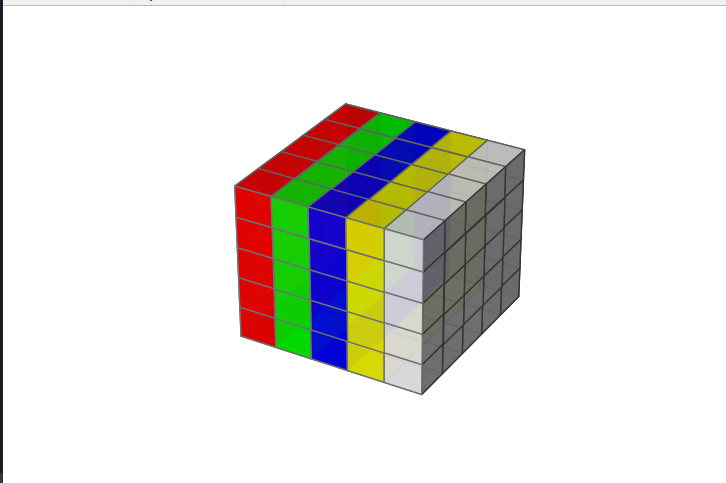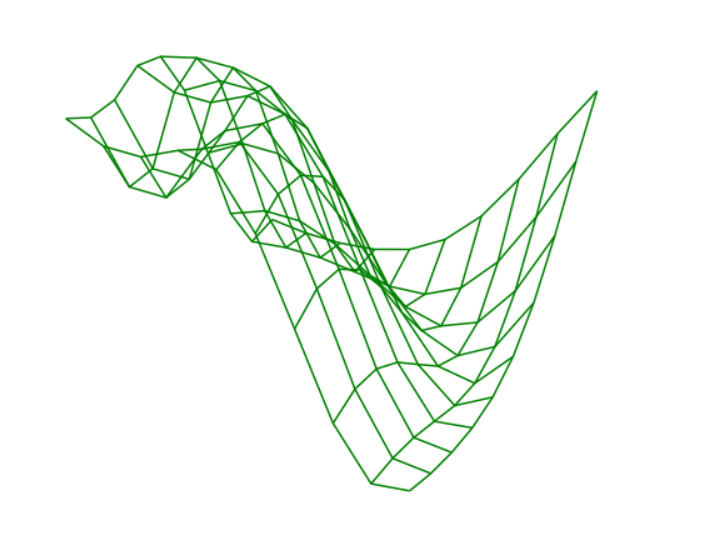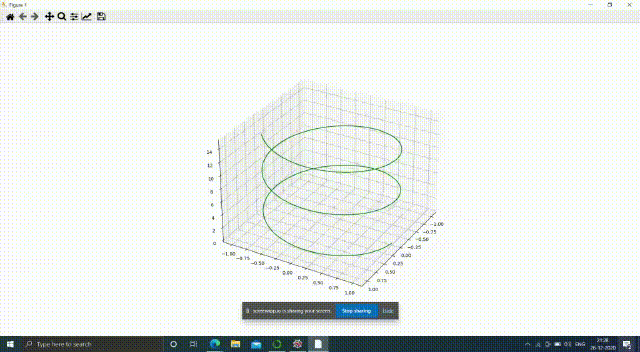Related Articles

# Displaying 3D images in Python

• Last Updated : 06 Jul, 2021

In this article, we will discuss how to display 3D images using different methods, (i.e 3d projection, view_init() method, and using a loop) in Python.

### Module Needed

• Matplotlib: It is a plotting library for Python programming it serves as a visualization utility library, Matplotlib is built on NumPy arrays, and designed to work with the broader SciPy stack.
• Numpy: It is a general-purpose array-processing package. It provides a high-performance multidimensional array and matrices along with a large collection of high-level mathematical functions.
• mpl_toolkits: It provides some basic 3d plotting (scatter, surf, line, mesh) tools.

Example 1:

In this example, we created a 3d image of a scatter sin wave. Here we have created an array of points using ‘np.arrange’ and ‘np.sin’.NumPy.sin: This mathematical function helps the user to calculate trigonometric sine for all x(being the array elements), and another function is the scatter() method which is the matplotlib library used to draw a scatter plot.

Syntax: np.arrange(start, stop, step) : It returns an array with evenly spaced elements as per the interval.

Parameters:

• start: start of interval range.
• stop: end of interval range.
• step: step size of the interval.

## Python3

 `# Import libraries``import` `numpy as np``import` `matplotlib.pyplot as plt``from` `mpl_toolkits.mplot3d ``import` `Axes3D` `# Change the Size of Graph using``# Figsize``fig ``=` `plt.figure(figsize``=``(``10``, ``10``))` `# Generating a 3D sine wave``ax ``=` `plt.axes(projection``=``'3d'``)` `# Creating array points using``# numpy``x ``=` `np.arange(``0``, ``20``, ``0.1``)``y ``=` `np.sin(x)``z ``=` `y``*``np.sin(x)``c ``=` `x ``+` `y` `# To create a scatter graph``ax.scatter(x, y, z, c``=``c)` `# trun off/on axis``plt.axis(``'off'``)` `# show the graph``plt.show()`

Output:Example 2:

In this example, we are selecting the 3D axis of the dimension X =5, Y=5, Z=5, and in np.ones() we are passing the dimensions of the cube. The np.ones () function returns a new array of given shape and type, with ones.

Syntax: numpy.ones (shape, dtype = None)

After the above step, we are selecting color opacity as alpha = 0.9 ( vary from 0.0 – 1.0 ). In the next step, we are passing the dimension of axes( i.e 5, 5, 5) + number of faces for the cube ( i.e 0-4 ) in np.empty() function after that we are passing color combination and opacity for each face of the cube and in last Voxels is used to customizations of the sizes, positions, and colors. The np.empty () function return a new array of given shape and type, without initializing entries.

Syntax: numpy.empty(shape)

## Python3

 `# Import libraries``import` `matplotlib.pyplot as plt``from` `mpl_toolkits.mplot3d ``import` `Axes3D``import` `numpy as np` `# Change the Size of Graph using``# Figsize``fig ``=` `plt.figure(figsize``=``(``10``, ``10``))` `# Generating a 3D sine wave``ax ``=` `plt.axes(projection``=``'3d'``)` `# Create axis``axes ``=` `[``5``, ``5``, ``5``]` `# Create Data``data ``=` `np.ones(axes)` `# Controll Tranperency``alpha ``=` `0.9` `# Control colour``colors ``=` `np.empty(axes ``+` `[``4``])` `colors[``0``] ``=` `[``1``, ``0``, ``0``, alpha]  ``# red``colors[``1``] ``=` `[``0``, ``1``, ``0``, alpha]  ``# green``colors[``2``] ``=` `[``0``, ``0``, ``1``, alpha]  ``# blue``colors[``3``] ``=` `[``1``, ``1``, ``0``, alpha]  ``# yellow``colors[``4``] ``=` `[``1``, ``1``, ``1``, alpha]  ``# grey` `# trun off/on axis``plt.axis(``'off'``)` `# Voxels is used to customizations of``# the sizes, positions and colors.``ax.voxels(data, facecolors``=``colors, edgecolors``=``'grey'``)`

Output:Example 3:

In this example, we use numpy.linspace() that creates an array of 10 linearly placed elements between -1 and 5, both inclusive after that the mesh grid function returns two 2-dimensional arrays, After that in order to visualize an image of 3D wireframe we require passing coordinates of X, Y, Z, color(optional).

## Python3

 `#Import libraries``import` `numpy as np``import` `matplotlib.pyplot as plt``from` `mpl_toolkits.mplot3d ``import` `Axes3D` `#Change the Size of Graph using Figsize``fig ``=` `plt.figure(figsize``=``(``10``,``10``))` `#Generating a 3D sine wave``ax ``=` `plt.axes(projection``=``'3d'``)`  `# assigning coordinates``x ``=` `np.linspace(``-``1``, ``5``, ``10``)``y ``=` `np.linspace(``-``1``, ``5``, ``10``)``X, Y ``=` `np.meshgrid(x, y)``Z ``=` `np.sin(np.sqrt(X ``*``*` `2` `+` `Y ``*``*` `2``))` `# creating the visualization``ax.plot_wireframe(X, Y, Z, color ``=``'green'``)` `# trun off/on axis``plt.axis(``'off'``)`

Output:Example 4:

In this example, we plot a spiral graph, and we will see its 360-degree view using a loop. Here, view_init(elev=, azim=)This can be used to rotate the axes programmatically.‘elev’ stores the elevation angle in the z plane. ‘azim’ stores the azimuth angle in the x,y plane.D constructor. The draw() function in pyplot module of the matplotlib library is used to redraw the current figure with a pause of 0.001-time interval.

## Python3

 `from` `numpy ``import` `linspace``import` `numpy as np``import` `matplotlib.pyplot as plt``from` `mpl_toolkits ``import` `mplot3d`  `# Creating 3D figure``fig ``=` `plt.figure(figsize``=``(``8``, ``8``))``ax ``=` `plt.axes(projection``=``'3d'``)` `# Creating Dataset``z ``=` `np.linspace(``0``, ``15``, ``1000``)``x ``=` `np.sin(z)``y ``=` `np.cos(z)``ax.plot3D(x, y, z, ``'green'``)` `# 360 Degree view``for` `angle ``in` `range``(``0``, ``360``):``    ``ax.view_init(angle, ``30``)``    ``plt.draw()``    ``plt.pause(.``001``)` `plt.show()`

Output:Attention geek! Strengthen your foundations with the Python Programming Foundation Course and learn the basics.

To begin with, your interview preparations Enhance your Data Structures concepts with the Python DS Course. And to begin with your Machine Learning Journey, join the Machine Learning – Basic Level Course

My Personal Notes arrow_drop_up